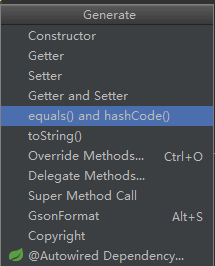# Lombok 注解 @Data 引出的 equals、==、hashCode

## Lombok 注解 @Data

``````@Data
public class User {
private String id;
private String name;

public User(String id, String name) {
this.id = id;
this.name = name;
}

}
``````

``````  public static void main(String[] args) {
User user1 = new User("1", "haier");
User user2 = new User("1", "haier");
System.out.println(user1 == user2);
System.out.println(user1.equals(user2));
}
``````

``````false
true
``````

``````    * @see     #hashCode()
* @see     java.util.HashMap
*/
public boolean equals(Object obj) {
return (this == obj);
}
``````

## equals、==、hashCode

``````public boolean equals(Object anObject) {
if (this == anObject) {
return true;
}
if (anObject instanceof String) {
String anotherString = (String)anObject;
int n = value.length;
if (n == anotherString.value.length) {
char v1[] = value;
char v2[] = anotherString.value;
int i = 0;
while (n-- != 0) {
if (v1[i] != v2[i])
return false;
i++;
}
return true;
}
}
return false;
}
``````

``````user1.hashCode();
``````

``````public native int hashCode();
``````

``````public int hashCode() {
int h = hash;
if (h == 0 && value.length > 0) {
char val[] = value;

for (int i = 0; i < value.length; i++) {
h = 31 * h + val[i];
}
hash = h;
}
return h;
}
``````

`````` s*31^(n-1) + s*31^(n-2) + ... + s[n-1]
``````

ok，再回头看第一部分例子就显得很奇怪。

``````		User user1 = new User("1", "haier");
User user2 = new User("1", "haier");
System.out.println(user1 == user2);
System.out.println(user1.equals(user2));
``````

`@EqualsAndHashCode`：用在类上，自动生成`equals`方法和`hashCode`方法``````@Override
public boolean equals(Object o) {
if (this == o) return true;
if (o == null || getClass() != o.getClass()) return false;
if (!super.equals(o)) return false;

User user = (User) o;

if (id != null ? !id.equals(user.id) : user.id != null) return false;
return name != null ? name.equals(user.name) : user.name == null;
}
``````

ok，完结。

## 《Lombok 注解 @Data 引出的 equals、==、hashCode》有1个想法

1.程序员

也就是字符串在堆内存空间中的值，而不是这个值的地址。 这句话不对吧
if (this == anObject) {
return true;
}
这个就是比较地址

回复